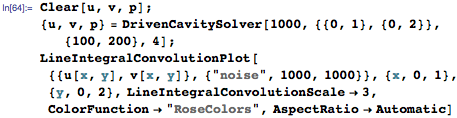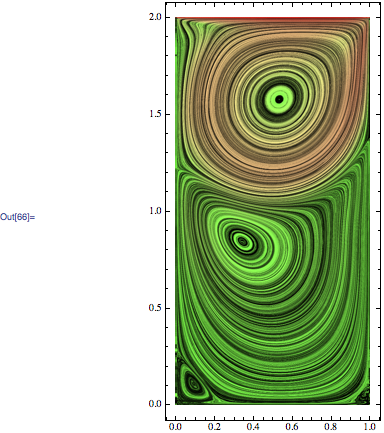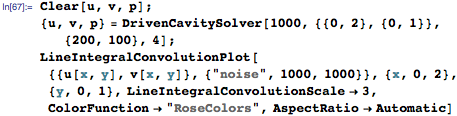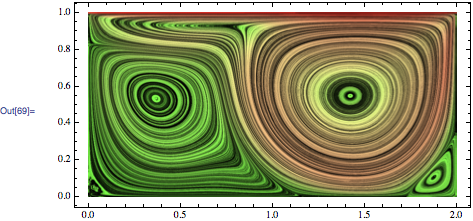Browse by Topic
Related Topics

# Using Mathematica to Simulate and Visualize Fluid Flow in a Box

The motion of fluid flow has captured the interest of philosophers and scientists for a long time. Leonardo da Vinci made several sketches of the motion of fluid and made a number of observations about how water and air behave. He often observed that water had a swirling motion, sometimes big and sometimes small, as shown in the sketch below.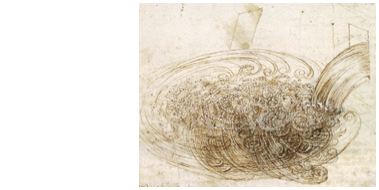We would now call such swirling motions vortices, and we have a systematic way of understanding the behavior of fluids through the Navier–Stokes equations. Let’s first start with understanding these equations.

We have all seen special effects and visualizations of fluids in movies. The special effects are meant to give the viewer a realistic look at the motion of water or air. Since the focus in movies is on aesthetics, the flow may not be accurate. So, how does one go about writing code that will simulate fluid flow? In general, the answer is quite complicated, but with a few assumptions can be made more tractable.

Assuming that the simulation will take place under normal conditions, that is, at a reasonable temperature and at sea level, the equations that govern the motion of a fluid are: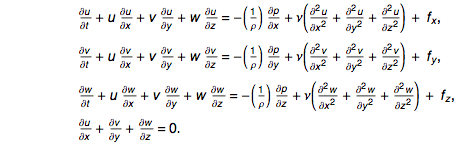These represent a time-dependent system of partial differential equations (or PDEs, for short) that can be solved with appropriate boundary conditions. u (m/s) , v (m/s), and w (m/s) represent the different components of the velocity; p (N/m2) represents the pressure; ν (m2/s) is the viscosity of the fluid; and ρ (kg/m3) is the density of the fluid. Fluids obeying this system of PDEs are called incompressible fluids.

Solving the above system in general is difficult. Many people might decide at this point that the problem is much too complicated, and they don’t have the time or expertise to write specialized code to do this. This may have been true in the past, but with Mathematica, the simulation can be done using a number of built-in functions and visualization in a couple of lines.

In this blog post, we”ll look at some techniques to help in simulating fluid flow and we’ll visualize the motion of the flow.

Even though the problem seems intimidating, a lot of information can be gathered by just looking at the system. Let’s use an understandable example. Consider a square box where the top lid is allowed to move in the horizontal plane. When the top lid is not moving, the air (or fluid) inside is stationary. However, when the lid starts moving, the motion of the lid makes the fluid circulate inside the box. Now, even without understanding how the flow will move, we can make a qualitative guess about what might be happening inside the box. Here is a sketch of that.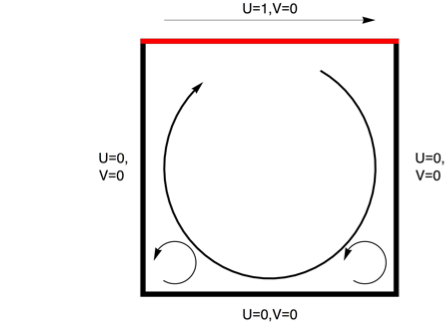The top lid drags the fluid below it, which generates a swirling motion. This initial swirling motion can instigate its own swirling motion, like a big gear turning smaller gears.

For the flow in a box, the Navier–Stokes equations can be simplified. First, we assume that the flow is not changing in time, and second, that it is not changing in the z direction. This simplifies the equations to: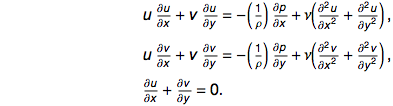This leads to three unknowns and three equations. If the velocities, pressure, and coordinates are non-dimensionalized as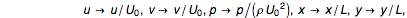where a characteristic velocity U0(m/s) and a characteristic length L (m) are used, the equations become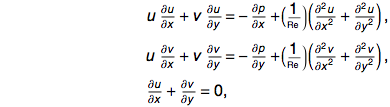where Re is called the Reynolds number and is defined as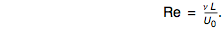As it turns out, the behavior of a flow can be described, to a large extent, by just this one number. The non-dimensionalization helps generalize the problem. So now the box can be made as large or as small as desired, and the top lid can be made to move fast or slow simply by changing the Reynolds number. This number can also be used to describe whether the fluid inside the box is water, air, or any other substance of your choice.

The Reynolds number is a very useful quantity in the field of fluid mechanics. This number can also be used to compare and characterize different flows. What does this mean? Suppose an engineer wanted to test the performance of a Boeing 747. It would be highly impractical (not to mention expensive) to run tests on the real airplane. The engineer can instead perform the tests on a model airplane with the Reynolds number of the model and the actual plane kept the same. This is the basis on which all wind-tunnel experiments are performed.

Now, back to the problem at hand. How can one solve the above system? As of now, there are no known analytical solutions, so the only choice is to obtain a solution using numerical methods.

The challenge in obtaining a numerical solution is to figure out the treatment of the derivatives. The general idea/algorithm is as follows:

1) Take the domain and discretize it. This means that the solution u(x) is computed at discrete points xi, and the solution is to be found at u(xi).

2) The derivatives are expressed as some linear combination of u(xi).

3) The final system is changed from a system of differential equations to a system of algebraic equations for u(xi). These can be solved using one of many root finding algorithms.

Let’s follow the algorithm outlined above. The first step is to discretize the domain. This means that we have to define a set of grid points on which the solution will be found. This is done as: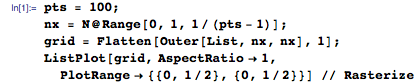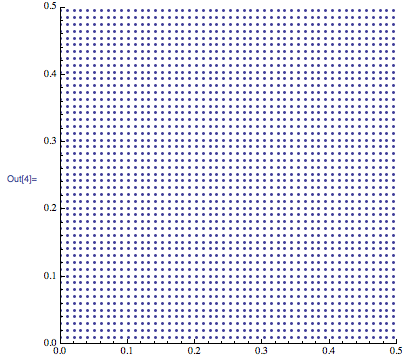At each of these grid points, the velocities u(xi, yi) and v(xi, yi) and pressure p(xi, yi) have to be computed. This means that each point contains three unknowns. We can give each unknown a name.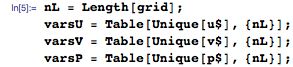The second step in the algorithm is to find an approximation to the derivatives. The derivatives need to be approximated as: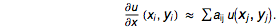This form of approximation is called finite difference approximation. The coefficients can be computed using the traditional Taylor series. NDSolve has a utility function called NDSolve`FiniteDifferenceDerivative that automatically computes these coefficients. Here is an example: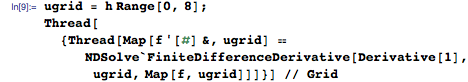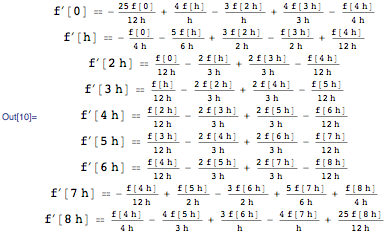The function NDSolve`FiniteDifferenceDerivative can also be used to get the result in a matrix form. Having a matrix representation would make it easier to code. As an example, let’s get the first-order derivative for Sin(x) where x ∈(0,1).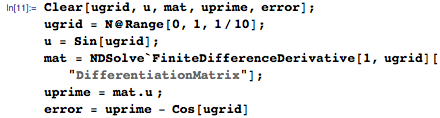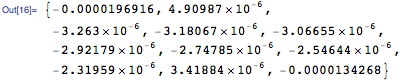So, with the help of NDSolve`FiniteDifferenceDerivative, the system of PDEs describing the flow can be converted to a system of nonlinear algebraic equations. For discretization, we need the differentiation matrices for the first and second derivatives in x and y. The accuracy of the finite difference approximation can be specified by the option “DifferenceOrder“. A value of 4 would mean that the approximation is 4th-order accurate.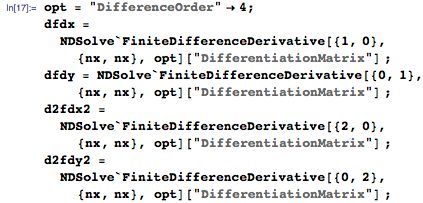With the variables defined and the matrices computed, the nonlinear algebraic equations are constructed as: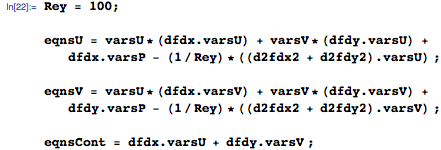Notice that the Reynolds number is taken to be 100 (this can, of course, be changed).

There is one additional step (which turns out to be an important step) to account for the boundary conditions. To do this, we need to find the grid points that lie on the boundary AND the equation numbers associated with them. This can done as: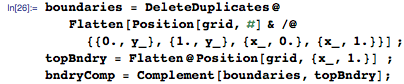Now that the boundary positions are known, the following boundary conditions need to be applied: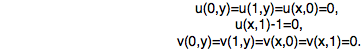Since the equation numbers associated with the boundaries are known, incorporating the boundary conditions involves a replacement of the discretized governing equations with the boundary equations.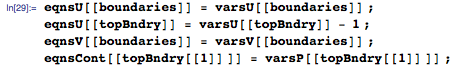With the discretization complete, we can proceed to solving the system. To do this, all the discretized equations should be joined into one large system of equations. This means that the final system to be solved will be 3n where n is the number of grid points. The 3 comes from the fact that we are solving three unknown variables at each grid point. So for a 100 x 100 grid, we will simultaneously solve 30,000 equations.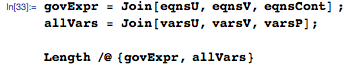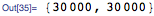The function FindRoot can be used to solve this system.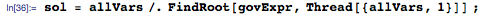Remember that the velocities are computed at discrete points. Using the function Interpolation, the discrete solution can be converted into a black-box continuous function.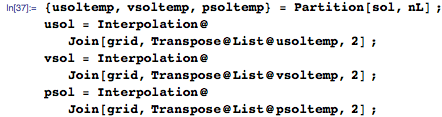We can visualize the interpolation using LineIntegralConvolutionPlot, which generates very attractive flow visualizations.

>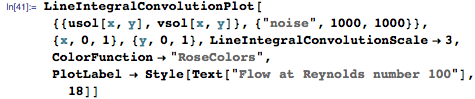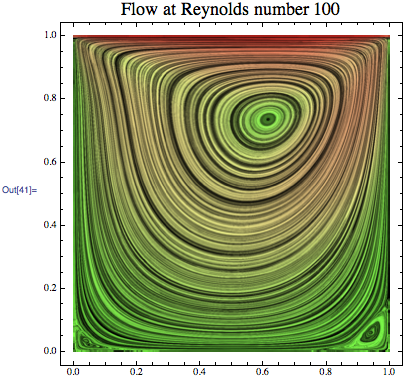We can construct a number of helper/utility functions in order to put the code in a reusable form.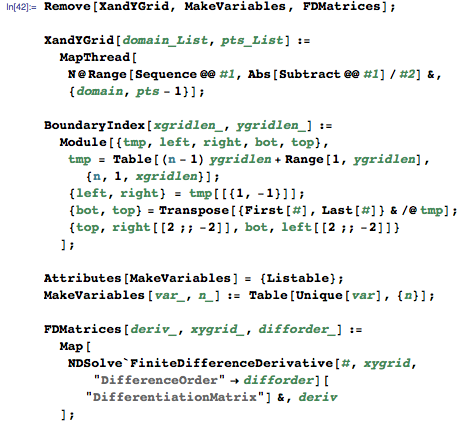With the help of the utility functions, the main solver function is relatively compact.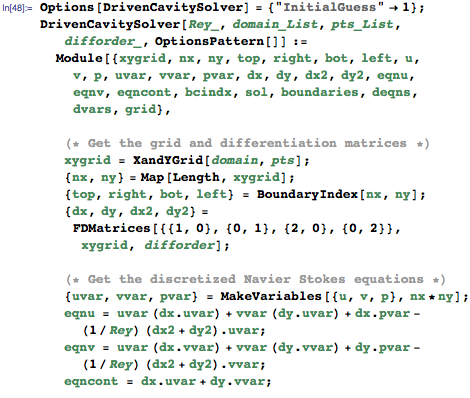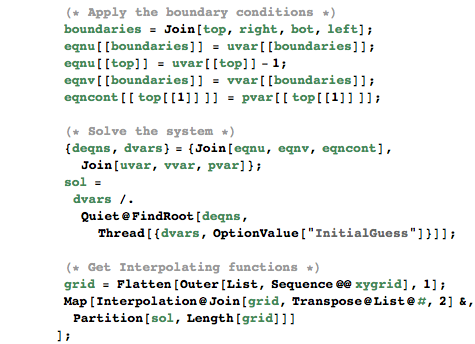The main solver function now consists of five parts. The functions of each of these parts are described as comments. Note that the main solver function “DrivenCavitySolver” takes in four arguments. These arguments are: Reynolds number, the domain, grid points in each direction, and the differentiation order. Having it in this form makes it easy to play with code. For example, the aspect ratio of the box can be changed to make it narrow or wide, and we can see what happens to the flow as a result of that change.

With the function now in hand, a number of interesting questions can be answered and different tests can be performed. The most obvious question that one can ask is: “What happens to the flow when the Reynolds number is increased?” Here are some results that easily answer this question through simulation and visualization.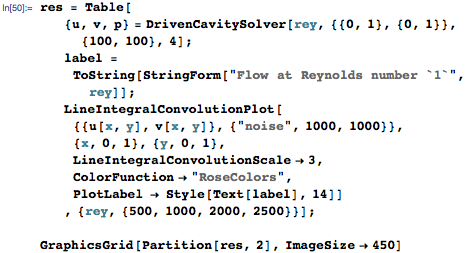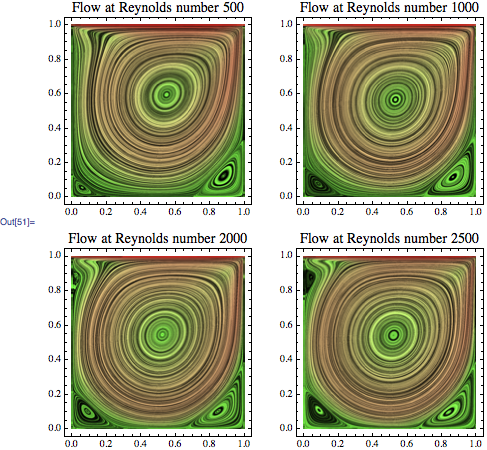Comparing the four flows, the common characteristic is a large central vortex dominating the flow. The differences between the four flows are seen in the secondary vortices. As the Reynolds number increases, the bottom-left vortex starts increasing in size, and eventually, when the Reynolds number is high enough, a third vortex starts forming at the top-left of the box (look at the fourth plot). We can therefore suspect the presence of more vortices as the Reynolds number is increased.

What we are viewing is a steady-state flow, which means that the flow does not change from one point in time to the next. This doesn’t mean that the flow is not moving! To visualize the actual motion of the flow, the box can be seeded with mass-less particles, and the particles are tracked in time as they are carried by the flow. This again can be done very easily using NDSolve.

The first step is to generate a flow. Let’s choose the flow at Reynolds number 2,000. This simulation will take a couple of minutes.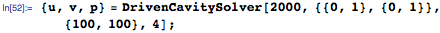The box is seeded with 400 particles equally spaced throughout the box. The results of tracking the trajectories of each of these particles are called pathlines.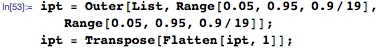Using NDSolve, each individual particle can be tracked as it is carried by the flow. Let’s select a time window of 10 time-units to see how the particles move during that time period.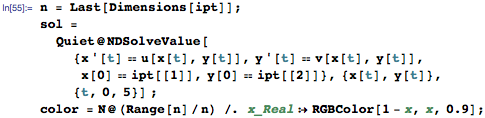The evolution of the particles can now be visualized using the function ListAnimate.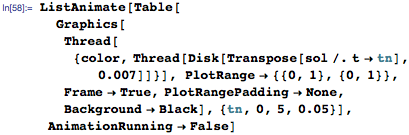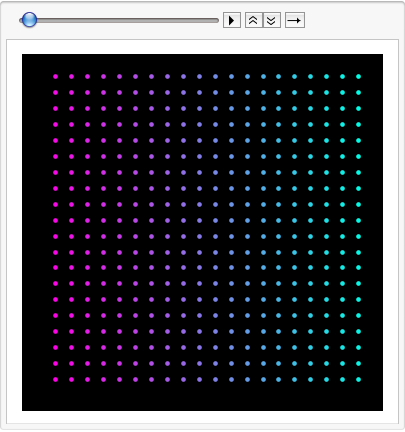Another idea for visualization is to release a continuous stream of dye at one location in space for a short period of time. The location can be determined by the experimenter. We will choose the location to be near the bottom-right of the box. A simple modification to the equations can achieve this effect.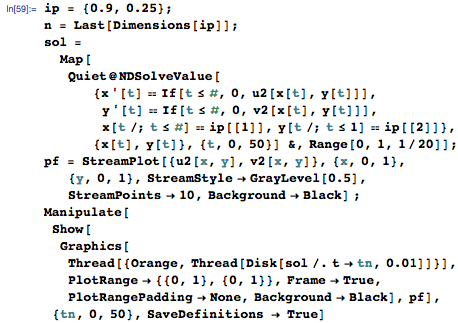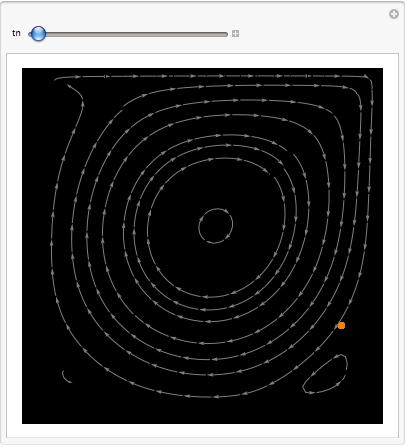Let’s experiment a little bit more. What happens when the aspect ratio is changed? If the aspect ratio is 2, then the movement of the primary vortex generates a second major vortex below it. As the strength of the primary vortex increases, it will tend to push the secondary vortex toward the left wall. Notice that if there is enough strength in this secondary vortex, it will generate its own vortex—the vortex at the bottom-left of the box.